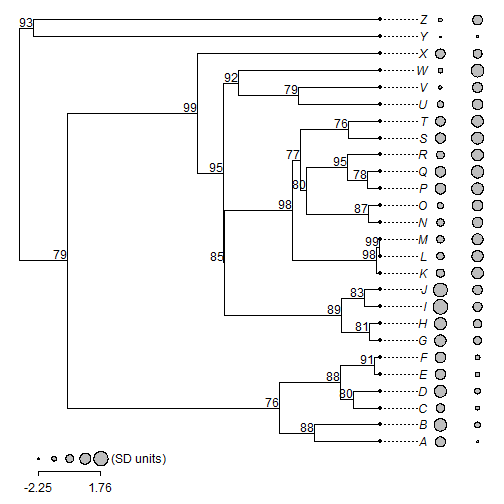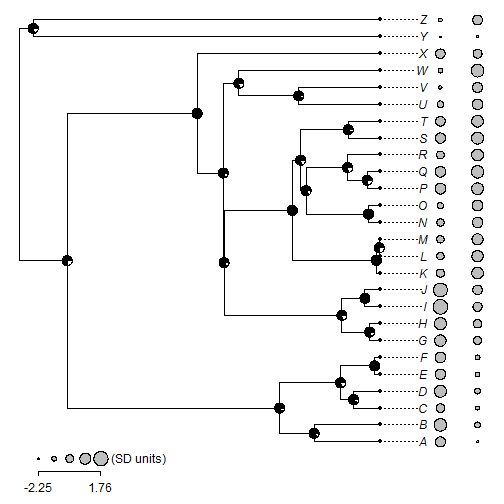## Wednesday, February 24, 2016

### Adding node labels (including bootstrap values) to a tree plotted using `dotTree`

Thank you very much for this great addition. May I ask if there's any way to add bootstrap value by each node on the tree plotted by dotTree?

The answer is “yes.” The internal plotting function used by `dotTree` (`phylogram`), though not in the namespace of phytools, nonetheless works in similar ways to the S3 plotting method `plot.phylo` in ape, and other phytools plotting functions such as `plotTree`, in that it sets the environmental variable `"last_plot.phylo"` & thus can be used with functions such as `nodelabels` and `tiplabels` from the ape package.

Here's a demo which assumes that the bootstrap values are stored as node labels on the object of class `"phylo"`:

``````library(phytools)
tree\$node.label
``````
``````##   ""   "76" "88" "88" "80" "91" "99" "95" "85" "89" "81" "83" "98" "98"
##  "99" "77" "80" "87" "95" "78" "76" "92" "79" "93" "79"
``````
``````dotTree(tree,X,standardize=TRUE,colors="grey")
nodelabels(tree\$node.label,node=2:tree\$Nnode+Ntip(tree),
``````Here, I am going to show the bootstrap proportion as a pie chart at each node:

``````dotTree(tree,X,standardize=TRUE,colors="grey")
nodelabels(node=1:tree\$Nnode+Ntip(tree),
pie=cbind(as.numeric(tree\$node.label),100-as.numeric(tree\$node.label)),
piecol=c("black","white"),cex=0.5)
``````Note that we have to be very careful with things like bootstrap values & posterior probabilities in R because though they are usually stored as node labels, they are actually associted with edges (i.e., splits) not with nodes! This distinction become important if we re-root the tree. Perhaps there should be more on this topic in a future post.

Data for this demo were simulated as follows:

``````tree<-pbtree(n=26,tip.label=LETTERS) ## random tree
X<-fastBM(tree,nsim=2) ## random traits
## random bootstraps
tree\$node.label<-c("",round(runif(n=tree\$Nnode-1,min=75,max=100)))
``````

1.Thank you for a fantastic package! I'm wondering how to label only specific nodes on a phylogeny based on the labels from the function nodelabels()? I'm interested in only labeling bootstrap support for the deeper nodes in my phylogeny and have a vector of nodelabels I'm interested in but am unsure how to access the bootstrap support for those labels. For example :
how would I access the bootstrap support in t\$tip.label for nodes labeled : c(1093, 1073, 1068)?

node = c(1093, 1073, 1068))

Thank you!

1.Hi Katie. Why are you bootstrap support values stored in the tip labels? Normally I would expect them to be stored as node labels. In which case you should be able to do something like:

nodes<-c(1093,1073,1068)
bs<-tree\$node.label[nodes-tree\$Nnode]
nodelabels(bs,nodes,...)

in which ... are the other arguments you want to send to nodelabels.

Let me know if this gets you on the right track.

- Liam

2.Excellent, thank you! Yes, my bootstrap support values were stored as node labels. In the future, is there a place where node numbers are stored? Is there a way to access them other than visualizing them on the tree with the nodelabels function? Thank you!

1.They should be in the vector tree\$node.label.

All the best, Liam

3.It is really a great work and the way in which you are sharing the knowledge is excellent.Thanks for your informative article

web designing course in chennai

4.This comment has been removed by the author.

5.I am new to this and would like to know how to call a tree constructed using Mega6.0 to R, and also to add bootstraps

6.Hi Liam,

I just saw this post. Is there a typo on the script of the first tree? Should it say "node=1:tree\$Nnode+Ntip(tree)" instead of "node=2:tree\$Nnode+Ntip(tree)" ?
Or should it say node=2:... for the script of the second tree?

I ask because there is a mismatch between the values in tree one and the pies in tree two.

Thanks!

Julia

Note: due to the very large amount of spam, all comments are now automatically submitted for moderation.